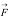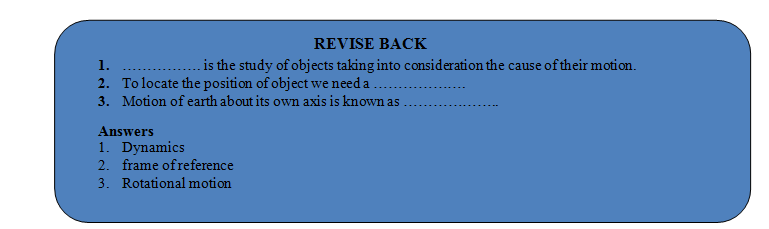# PHYSICAL QUANTITIES

## MOTIONS IN ONE, TWO AND THREE DIMENSIONS (TYPE OF MOTION)

As position of the object may change with time due to change in one or two or all the three coordinates, so we have classified motion as follows:

### MOTION IN ONE DIMENSION:

If only one of the three co-ordinates specifying the position of object changes w.r.t. time. In such a case the object moves along a straight line and the motion therefore is also known as rectilinear or linear motion.

Ex.

•  Motion of train along straight railway track.
•  An object falling freely under gravity.
•  When a particle moves from P1 to P2 along a straight line path only the x-co-ordinate changes.

### MOTION IN TWO DIMENSION:

If two of the three co-ordinates specifying the position of object changes w.r.t. time, then the motion of object is called two dimensional. In such a motion the object moves in a plane.

Ex.

• Motion of queen on carom board.
• An insect crawling on the floor of the room.
• Motion of object in horizontal and vertical circles etc.
• Motion of planets around the sun.
• A car moving along a zigzag path on a level road.

### MOTION IS THREE DIMENSION:

If all the three co-ordinates specifying the position of object changes w.r.t. time, then the motion of object is called 3-D. In such a motion the object moves in a space.

Ex.

• A bird flying in the sky (also kite).
• Random motion of gas molecules.
• Motion of an aeroplane in space.

### TYPES OF MOTION

• Linear motion (or translatory motion) : The motion of a moving car, a person running, a stone being dropped.
• Rotational motion : The motion of an electric fan, motion of earth about its own axis.
• Oscillatory motion : The motion of a simple pendulum, a body suspended from a spring (also called to and fro motion).

### PHYSICAL QUANTITIES

Those quantities which can be measured are known as physical quantities. For example, mass, weight, speed, velocity, acceleration etc.

### TYPES OF PHYSICAL QUANTITIES:

• Scalar Quantities
• Vector Quantities

Scalar Quantities: The physical quantities which are completely described by the magnitude only are known as scalar quantities. Mass, length, time, work, energy, power etc. are scalar quantities.

Vector Quantities: The physical quantities which are completely described by the magnitude as well as the direction are known as vector quantities. Velocity, acceleration, weight, displacement etc, are vector quantities.

A vector is represented by a letter (symbol) having arrow over it. e.g..

Where F denotes the force.

### DIFFERENCE BETWEEN SCALAR AND VECTOR QUANTITIES:

 S. No. Scalar quantities Vector quantities 1. Scalar quantities are described by magnitude only. Vector quantities are described by magnitude as well as direction. 2. Scalar quantities change with change in magnitude only. Vector quantities change with the change in magnitude or with the change in direction or both. 3. Scalar quantities can be added or subtracted using ordinary rules of algebra. Vector quantities cannot be added or subtracted using ordinary rules of algebra. 4. Scalar quantities are represented by ordinary letter.  e.g. A Vector quantities are represented by letters having arrow over them. e.g..